Create a new printableMath Worksheets

Sample - Click above to make a new math worksheet (PDF).
 Name _____________________________Date ___________________
1.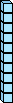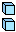-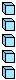12  -  7  =  ___
2.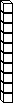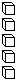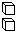-17  -  10  =  ___
3.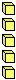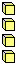+9  +  4  =  ___

Fill in the missing numbers
1.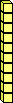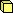- ___ =16  -  ___  =  10
2.- ___ =15  -  ___  =  7
3.
 ___ +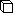=___  +  6  =  12

Fill in the missing numbers
1.+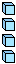___  +  ___  =  ___
2.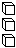-___  -  ___  =  ___

 Key #2
 aKey #2
Write the sum or difference.
1.
 4 + 9
2.
 17 - 8
3.
 2 + 10
4.
 15 - 7
5.
 9 + 7
6.
 11 - 4
7.
 19 - 9
8.
 7 + 10
9.
 16 - 6
10.
 1 + 10

Write the sum or difference.
 1 5  +  9  =  _____
 2 19  -  10  =  _____
 3 1  +  10  =  _____
 4 12  -  6  =  _____
 5 14  -  5  =  _____
 6 4  +  7  =  _____

Fill in the missing numbers.
1.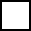- 9 7
2.
 14 -7
3.+ 10 16
4.
 2 +12
5.- 9 6

 Key #2
 aKey #2
Write the sum or difference.
 1 3  +  10  =  _____
 2 12  -  6  =  _____
 3 9  +  9  =  _____
 4 14  -  8  =  _____
 5 2  +  10  =  _____
 6 6  +  7  =  _____

Write an addition or subtraction sentence to solve each word problem.
1.
 Sarah has 8 pink dresses and 5 blue dresses. How many dresses does she have in all? _____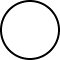_______________ dresses
2.
 Austin has a spelling test of 13 words. He misspelled 4 of them. How many words did he get right? ____________________ words

Write an addition or subtraction sentence to solve each word problem.
1.
 Madison had 18 boxes of cookies. She sold 9 of them. How many boxes are left? ____________________ boxes
2.
 Kaylee has 3 pink dresses and 8 blue dresses. How many dresses does she have in all? ____________________ dresses